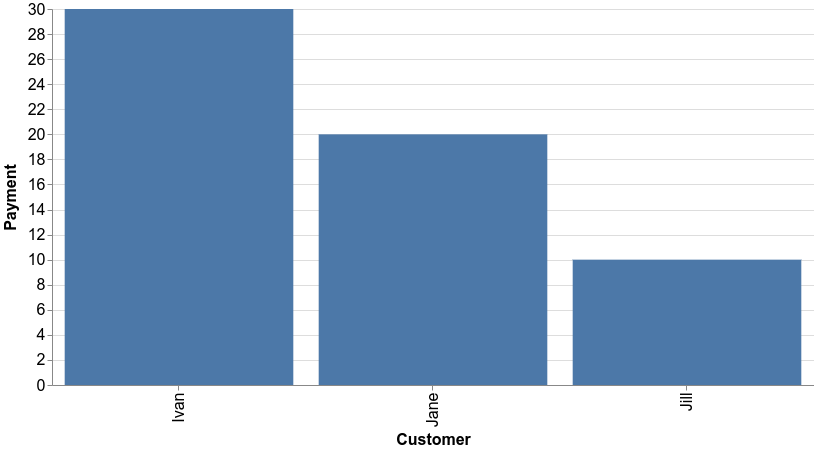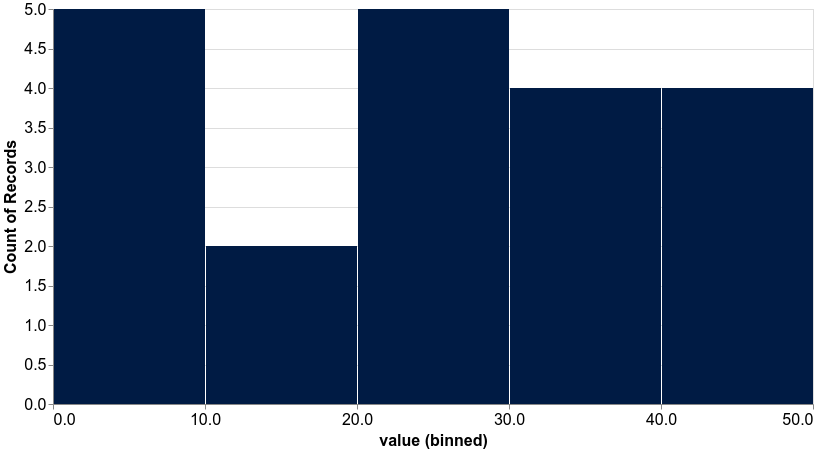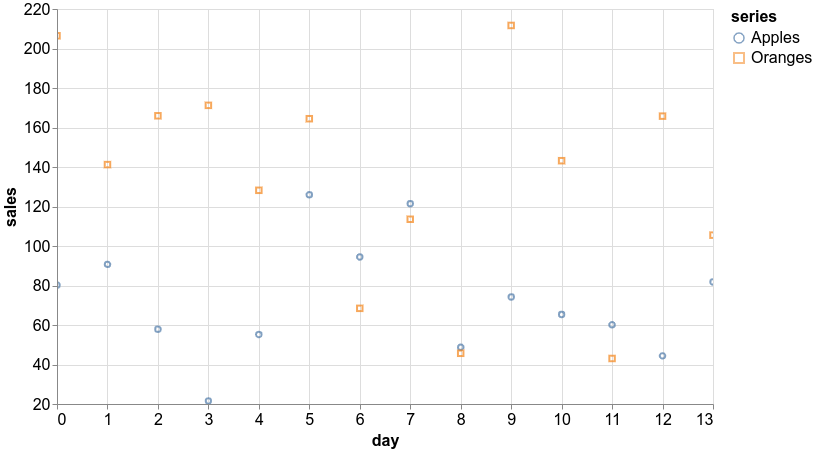# Curiousily

## Getting Started with TensorFlow.js | Deep Learning for JavaScript Hackers (Part 0)

Machine Learning, JavaScript, TensorFlow, Data Science3 min read

Share

TL;DR Learn about the basics of Machine Learning with TensorFlow.js - Tensors, basic visualizations and train a simple model that converts kilograms to pounds

So what is this thing TensorFlow.js?

TensorFlow.js is a library for developing and training ML models in JavaScript, and deploying in browser or on Node.js

For our purposes, TensorFlow.js will allow you to build Machine Learning models (especially Deep Neural Networks) that you can easily integrate with existing or new web apps. Think of your ReactJs, Vue, or Angular app enhanced with the power of Machine Learning models.

Run the complete source code for this tutorial right in your browser:

# Tensors

Tensors are the main building blocks of TensorFlow. They are n-dimensional data containers. You can think of them as multidimensional arrays in languages like PHP, JavaScript, and others. What that means is that you can use tensors as a scalar, vector, and matrix values, since they are a generalization of those.

Each Tensor contains the following properties

• rank - number of dimensions
• shape - size of each dimension
• dtype - data type of the values

Let’s start by creating your first Tensor:

1import * as tf from "@tensorflow/tfjs"23const t = tf.tensor([1, 2, 3])

Check it’s rank:

1console.log(t.rank)
11

That confirms that your Tensor is 1-dimensional. Let’s check the shape:

1console.log(t.shape)
1

1-dimensional with 3 values. But how can you see the contents of this thing?

1console.log(t)
1Tensor {kept: false, isDisposedInternal: false …}

Not what you’ve expected, right? Tensors are custom objects and have a print() method that output their values:

1t.print()
1Tensor2    [1, 2, 3]

Of course, the values don’t have to be just numeric. You can create tensors of strings:

1const st = tf.tensor(["hello", "world"])

You can use tensor2d() to create matrices (or 2-dimensional tensors):

1const t2d = tf.tensor2d([2  [1, 2, 3],3  [4, 5, 6],4])5console.log(t2d.shape)
1[2, 3]

There are some utility methods that will be handy when we start developing models. Let’s start with ones():

1tf.ones([3, 3]).print()
1Tensor2    [[1, 1, 1],3     [1, 1, 1],4     [1, 1, 1]]

You can use reshape() to change the dimensionality of a Tensor:

1tf.tensor([1, 2, 3, 4, 5, 6]).reshape([2, 3]).print()
1Tensor2    [[1, 2, 3],3     [4, 5, 6]]

## Tensor Math

1const a = tf.tensor([1, 2, 3])2const b = tf.tensor([4, 5, 6])34a.add(b).print()
1Tensor2    [5, 7, 9]

and dot() to compute the dot product of two tensors:

1const d1 = tf.tensor([2  [1, 2],3  [1, 2],4])5const d2 = tf.tensor([6  [3, 4],7  [3, 4],8])9d1.dot(d2).print()
1Tensor2    [[9, 12],3     [9, 12]]

Finally, let’s have a look at transpose():

1tf.tensor([2  [1, 2],3  [3, 4],4])5  .transpose()6  .print()
1Tensor2    [[1, 3],3     [2, 4]]

You can think of the transpose as a flipped-axis version of the input Tensor.

Have a look at all arithmetic operations

# Visualization with tfjs-vis

tfjs-vis is a small library for in browser visualization intended for use with TensorFlow.js.

Let’s start by creating a simple bar chart. Here’s what our data looks like:

1import * as tfvis from "@tensorflow/tfjs-vis"23const data = [4  { index: "Jill", value: 10 },5  { index: "Jane", value: 20 },6  { index: "Ivan", value: 30 },7]

Now, let’s render the data using barchart():

1const container = document.getElementById("barchart-cont")2tfvis.render.barchart(container, data, {3  xLabel: "Customer",4  yLabel: "Payment",5  height: 350,6  fontSize: 16,7})

Note that we provide a DOM element to the renderer as a container for our chart, which might be handy when you want to embed the charts in your apps.Let’s have a look at histogram() and create a sample chart:

1const data = Array(20)2  .fill(0)3  .map(x => Math.random() * 50)45const container = document.getElementById("histogram-cont")6tfvis.render.histogram(container, data, {7  maxBins: 5,8  height: 450,9  fontSize: 16,10})The API is pretty consistent for those 2 charts. Let’s do a scatter plot:

1const apples = Array(14)2  .fill(0)3  .map(y => Math.random() * 100 + Math.random() * 50)4  .map((y, x) => ({ x: x, y: y }))56const oranges = Array(14)7  .fill(0)8  .map(y => Math.random() * 100 + Math.random() * 150)9  .map((y, x) => ({ x: x, y: y }))1011const series = ["Apples", "Oranges"]1213const data = { values: [apples, oranges], series }1415const container = document.getElementById("scatter-cont")16tfvis.render.scatterplot(container, data, {17  xLabel: "day",18  yLabel: "sales",19  height: 450,20  zoomToFit: true,21  fontSize: 16,22})Have a look at the complete tfjs-vis API

Time to put what you’ve learned into practice and build your first model. To make it somewhat realistic, we’ll try to approximate the conversion of kgs to lbs, which is described by this function:

1const kgToLbs = kg => kg * 2.2

Let’s use it to prepare our data and create 2000 training examples:

1const xs = tf.tensor(Array.from({ length: 2000 }, (x, i) => i))2const ys = tf.tensor(Array.from({ length: 2000 }, (x, i) => kgToLbs(i)))

We’re going to use a style of Machine Learning known as Supervised Learning. In a nutshell, we need to provide 2 arrays to our model - X is the training features (kilograms), and y is the training labels (corresponding pounds).

TensorFlow.js allows you to build layered models using sequential(). We’re going to go extremely simple: 1 layer, input size of 1, and 1 learning parameter:

1const model = tf.sequential()23model.add(tf.layers.dense({ units: 1, inputShape: 1 }))

and teach it to convert kilograms to pounds:

1model.compile({2  loss: "meanSquaredError",3  optimizer: "adam",4})56await model.fit(xs, ys, {7  epochs: 100,8  shuffle: true,9})

Your model needs a metric to know how well is doing. In our case that is Mean Squared Error (MSE). Once you know how to measure the error, you need something to know how to minimize it using the data. In our case, that is the Adam optimizer.

Finally, we use the data to train our model for 100 epochs (number of times our model sees the data) and request to shuffle it. Why shuffle? We don’t want our model to learn the ordering of the data, just the relationship between different examples.

After the training is complete (might take some time) you can use your model to predict what amount of pounds correspond to 10 kg:

1const lbs = model2  .predict(tf.tensor())3  .asScalar()4  .dataSync()56console.log("10 kg to lbs: " + lbs)
110 kg to lbs: 22.481597900390625

Seems to be doing good, right?

# Conclusion

Congratulation on finishing the first part of your journey to Machine Learning understanding. You learned about:

• Tensors: n-dimensional data containers
• tfjs-vis: visualization library integrated with TensorFlow.js
• predict pounds from kilograms using a simple model

Run the complete source code for this tutorial right in your browser:

I hope that this tutorial just made you thirsty for knowledge about what is possible with Machine Learning and JavaScript. Ready for the next one?

Share

## Want to be a Machine Learning expert?

Join the weekly newsletter on Data Science, Deep Learning and Machine Learning in your inbox, curated by me! Chosen by 10,000+ Machine Learning practitioners. (There might be some exclusive content, too!)

You'll never get spam from me

© 2021 Curiousily by Venelin Valkov